Scientific Models

Part of a Teacher Institute workshop by Paul Doherty

"If it can't be expressed in figures it's not science it's opinion." R, Heinlien

A model of scientific models

To help make sense of the world scientists create models. These models are powerful tools to help scientists predict the results of experiments. Correctly predicting the result of an experiment is a test for the model, when a model fails to predict correctly an experimental result, the model must be revised or replaced.

Light

For example, Newton modeled light as a particle, but when Young showed that light produced interference patterns he changed the model to a wave, later still Einstein showed that light energy must be quantized to explain the photoelectric effect, today we model light as a quantized wave of the probability density function. In our current model, light is created or destroyed as a quanta of energy but travels as a wave. This dichotomy between real light and scientific models of light is well described by physics professor Brian Holmes who says, "Light is light, sometimes light behaves like a particle, sometimes light behaves like a wave."

It's more complicated than that

Scientists answer questions from other scientists with mathematical models, graphs, tables and drawings, accompanied by very precisely worded verbal arguments. Their answers must be so precise that they are often difficult to follow and take a long time to state.

Teachers, on the other hand, answer questions from students using the simplest models of science that work to answer each particular question. Students should keep in mind that such an answer always carries an asterisk* with it:

*It's more complicated than that.

Ask me a question, I will give you an answer based on the simplest scientific model I can use. If you then refine your question I may have to switch my answer to use a more complicated model.

Choosing the correct model to use in answering a science question is one of the most difficult things to do.

For example, if you ask me about the orbit of the moon I'll answer you by using Newton's laws, however if you ask me about two neutron stars orbiting each other I'll have to switch to Einstein's general relativistic model. There is no need to use general relativity in a high school class when discussing the orbit of the moon.

Here are examples of a few scientific models:

Atoms

An excellent example of a scientific model is the atomic theory. The atomic theory organizes a tremendous amount of chemical information in a simple statement: All ordinary matter is made of atoms, small indivisible particles which attract each other when a little ways apart and repel each other when close together. (this is Feynman's statement of the atomic theory) The atomic theory provides a conceptual basis for the periodic table and for thermodynamics.

The atomic theory is a useful model, independent of whether atoms actually exist.

At the beginning of the 20'th century there were scientists who believed that atoms did not exist, although many admitted that atoms provided a good model for explaining a lot of chemistry. It was Einstein and his mathematical description of Brownian motion who finally convinced many of them of the existence of atoms. Today, scanning tunneling microscopes produce images of atoms.

The Standard Model of Particles and Fields

The Standard Model of particles and fields provides an organizational framework for a tremendous amount of information. The organization is useful, it actually predicted several particles before they were found. It describes every known particle, there are no known particles that it doesn't describe. The standard model describes electromagnetic fields as well as the weak and strong nuclear forces, it does not describe gravity. One part of the Standard Model uses quarks as a constituent of protons and other elementary particles. No one has ever found a single quark by itself, some scientists wonder how much quarks can be thought to really exist, maybe they are just convenient models, as atoms were 100 years ago.

String Theory

String theory extends the standard model to allow it to describe gravity fields. At the moment the predictions of string theory cannot be tested. In the year 2000 string theory is in the same position as the atomic theory was in 1900. It organizes a great deal of information yet its predictions cannot be tested.

Using Models

Magnetism

An early model of magnetism was that there are two types of magnetic poles, north and south. Like poles repel each other and unlike poles attract. Iron is attracted to both north and south poles.

Try this exploration of magnetic poles.

This basic model is very quickly extended to note that the force between magnetic poles depends on the distance between them, near poles exert stronger forces than the same poles when they are at a greater distance.

There is a simple experiment that suggests a new model for magnetism. Place two disk magnets side-by-side, face down on a table. Slide them together and they will repel each other since their north poles are close together and like poles repel (similarly their south poles are together.) However as they approach, one edge of one magnet will rise up into the air and balance in that elevated position.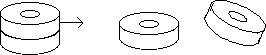Place two magnets one on top of the other. Slide the top magnet to one side and one edge will rise up into the air and hang in place against gravity.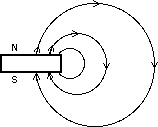To understand this behavior we need to model magnetic interactions through magnetic fields. Instead of saying north poles repel north poles we say instead that magnetic poles create magnetic fields which exert forces on magnetic poles.

Magnetic oscillators

To explain this behavior we use the model that magnets create a magnetic field and that this magnetic field exerts forces on other magnets. The magnetic field is usually portrayed by field lines. The field lines show the direction of the force on a lone magnetic north pole.

Models of Electric Force

Place the sticky side of one 10 cm (4 inch) long tape against the smooth side of another of equal length, then pull the tapes apart. Notice that the tapes attract each other.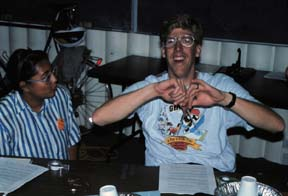A Paul Trudelle brings two pieces of electrically charged tape together and observes that they repel eacgh other.

Tape Electroscope

What's Going On?

When you pull the tapes apart one became positively charged while the other became negatively charged.

In the 1670 model of Newton the tapes would repel because of "action at a distance." This model predicts that if you move one tape the other will move instantly.

In the 1870 model of electromagnetism by Maxwell the tapes repel because the electric charges on one tape create an electric field throughout space. This electric field from one tape then exerts a force on the electric charges of the other tape. repelling it. It takes time for the changes in the electric field to propagate across space at the speed of light.

Tape Electromagnetic Wave

Polarized Light

Polarizer Model

Paul Hewitt uses the picket fence model of a light polarizer. In this model light is shown as a wave on a rope oscillating in the vertical direction as it travels along the rope. When this oscillating wave passes through a picket fence the wave passes through the fence.

Light waves oscillating perpendicular to the fence are absorbed.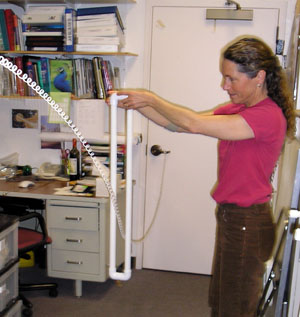Lori Lambertson holds a PVC model of a polarizer around a cord model of a vertically polarized light wave.

In real polarizers long chain polymers are coated with iodine. These coated polymers absorb light that is polarized parallel to the polymer chains and transmit light that is polarized perpendicular to the polymer chains.

Atomic Orbitals

Bohr came up with the model that electrons were sinusoidal waves that created quantized energy levels in an atom when an integer number of waves fit around the orbit.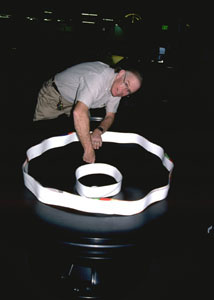Paul Doherty places circles with one complete wavelength and two complete wavelengths inside of a gravity well at a museum. The gravity well has the same shape as the electrostatic energy well in an atom.

Bohr Model for an atom

An even simpler model explores the gravitational energy level model of a stool.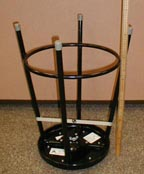A stool is in its ground state, where its center of mass, marked on the tape, is lowest.

Energy levels of a stool

Sinusoidal waves were a good start, but Schroedinger expanded this idea to include three dimensional shapes, the spherical Legendre Polynomials, which give rise to the atomic orbitals we learn in chemistry.

An excellent program that shows electron orbitals is

Models of Electricity

What is voltage? Which way does electrical current flow? Does it go from the positive side of a battery to the negative side or the other way around? We'll spend the rest of these web pages exploring these questions but before we begin experimenting, let me set you up for the answers.

Electricity

It is the same with electricity. In many activities, you are going to encounter models for voltage and current. Voltage and current are probably new things in your experience, they were new to Franklin, Volta, and Maxwell. Yet, in a very human way these people created models to describe voltage and current in more familiar terms. We will perform experiments then try to predict their outcomes and understand their results by employing models for electric current and voltage. Keep in mind that when we describe current as a flow of positive charges we are using a model meant to make thinking easier. Choosing the "right" model, the simplest one that gives a good enough answer, is part of the art of scientific explanation and teaching. Choosing the right model will become easier as you gain experience with electricity. Soon you might be describing the flow of fluid through pipes using a model in which fluid flow is compared to electrical current!

Current

Along these lines, current has been modeled as the flow of an electrical fluid, voltage was the pressure which drove that fluid. The "high-tension line" is a leftover from this model. The high tension is actually high-voltage and has nothing to do with the tightness of the stretch of the line. But even when you hear electric current being modeled as the flow of some fluid like water watch for places where the model breaks down, does electric current have inertia like water, is it incompressible? Watch for the places where models break down. Electric current is not exactly like the flow of water and its "inertia" or "compressibility" are quite different concepts from the ones we are used to applying to water.

In one model current is the flow of negative charges, in another it is the flow of positive charges. The actual electric current is more complicated than a simple flow of positive and negative particles and yet the simple model of current as a flow of one type of particles is usually good enough to provide the correct description of the results of an experiment. So we'll usually model electric current as the flow of positive particles, occasionally we'll model it as the flow of negatives, and rarely we'll switch back and forth between models.

Electrical engineers use the model of current as the flow of positive charges, high school science teachers often use the model of current as the flow of negative electrons.

The flow of electric current can be modeled by rolling small cylindrical magnets around the outside rim of a larger cylindrical magnet.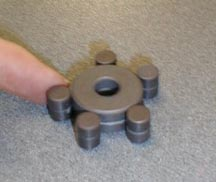A model for electric current. Roll small cylindrical magnets around the rim of a larger cylindrical magnet.

Magnets Round the Rim

Voltage

One model for voltage is that it is like height. So picture an electric circuit on a table, then visualize voltage at various points around the circuit as the height. The higher the voltage the higher the height. Just like height you need a reference point from which height is measured. This reference point on maps of the earth is called sea level. The reference point in your electric circuit is called ground or common. Voltage is the energy per electric charge, it is measured in volts per coulomb. Height is a measure of the energy per unit mass it is measured in meters or alternately in joules per kilogram. So height gives energy per unit mass and voltage gives energy per unit charge. They are similar.

The Waterfall Model

So, if you model one part of an electric circuit as a waterfall then a high waterfall models a high voltage difference, if a lot of water flows over this high fall, then it models a high voltage circuit with a lot of current. If a small amount of water goes over a high fall it models a high voltage circuit with a little current. A low waterfall models a low voltage circuit with either a tiny flow of water or a huge flow depending on the current

Conclusion

So. We'll use models to help us understand the new concepts of electricity. Remember that the model is not exact. Current is like the flow of a fluid in many, but not all, ways.

 Scientific Explorations with Paul Doherty © 2005 5 April 2005